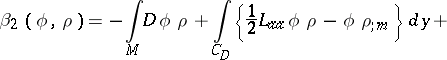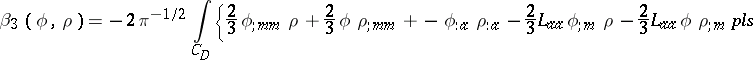# Heat content asymptotics

Let $M$ be a compact Riemannian manifold with boundary $\partial M$. Assume given a decomposition of the boundary as the disjoint union of two closed sets $C _ { N }$ and $C _ { D }$. Impose Neumann boundary conditions on $C _ { N }$ and Dirichlet boundary conditions on $C _ { D }$. Let $u _ { \Phi }$ be the temperature distribution of the manifold corresponding to an initial temperature $\Phi$; $u _ { \Phi } ( x ; t )$ is the solution to the equations:

\begin{equation*} ( \partial _ { t } + \Delta ) u = 0, \end{equation*}

\begin{equation*} u ( x ; 0 ) = \Phi ( x ) , u _ { ; m } ( y ; t ) = 0 \text { for } y \in C _ { N } , t > 0, \end{equation*}

\begin{equation*} u ( y ; t ) = 0 \text { for } y \in C _ { D } , t > 0. \end{equation*}

Here, $u_{:m}$ denotes differentiation with respect to the inward unit normal. Let $\rho$ be a smooth function giving the specific heat. The total heat energy content of $M$ is given by

\begin{equation*} \beta ( \phi , \rho ) ( t ) = \int _ { M } u _ { \Phi } \rho. \end{equation*}

As $t \downarrow 0$, there is an asymptotic expansion

\begin{equation*} \beta ( \phi , \rho ) ( t ) \sim \sum _ { n \geq 0 } \beta _ { n } ( \phi , \rho ) t ^ { n / 2 }. \end{equation*}

The coefficients $\beta _ { n } ( \phi , \rho )$ are the heat content asymptotics and are locally computable.

These coefficients were first studied with $C _ { N }$ empty and with $\phi = \rho = 1$. Planar regions with smooth boundaries were studied in [a5], [a6], the upper hemisphere was studied in [a4], [a3], and polygonal domains in the plane were studied in [a7]. See [a11], [a12] for recursive formulas on a general Riemannian manifold.

More generally, let $L$ be the second fundamental form and let $R$ be the Riemann curvature tensor. Let indices $a$, $b$, $c$ range from $1$ to $m - 1$ and index an orthonormal frame for the tangent bundle of the boundary. Let ":" (respectively, ";" ) denote covariant differentiation with respect to the Levi-Civita connection of $\partial M$ (respectively, of $M$) summed over repeated indices. The first few coefficients have the form:

$\beta _ { 0 } ( \phi , \rho ) = \int _ { M } \phi \rho$;

$\beta _ { 1 } ( \phi , \rho ) = - 2 \pi ^ { - 1 / 2 } \int _ { C _ { D } } \phi \rho$;\begin{equation*} + \int _ { C _ { N } } \phi _ { ; m } \rho \,d y; \end{equation*}\begin{equation*} + \frac { 4 } { 3 } \pi ^ { - 1 / 2 } \int _ { C _ { N } } \phi _ { ; m } \rho _ { ; m } d y. \end{equation*}

The coefficient $\beta _ { 4 }$ is known.

The coefficients $\beta_5$ and $\beta_6$ have been determined if $C _ { D }$ is empty.

One can replace the Laplace operator $\Delta$ by an arbitrary operator of Laplace type as the evolution operator [a1], [a2], [a10], [a9]. One can study non-minimal operators as the evolution operator, inhomogeneous boundary conditions, and time-dependent evolution operators of Laplace type. A survey of the field is given in [a8].

How to Cite This Entry:
Heat content asymptotics. Encyclopedia of Mathematics. URL: http://encyclopediaofmath.org/index.php?title=Heat_content_asymptotics&oldid=50826
This article was adapted from an original article by P.B. Gilkey (originator), which appeared in Encyclopedia of Mathematics - ISBN 1402006098. See original article